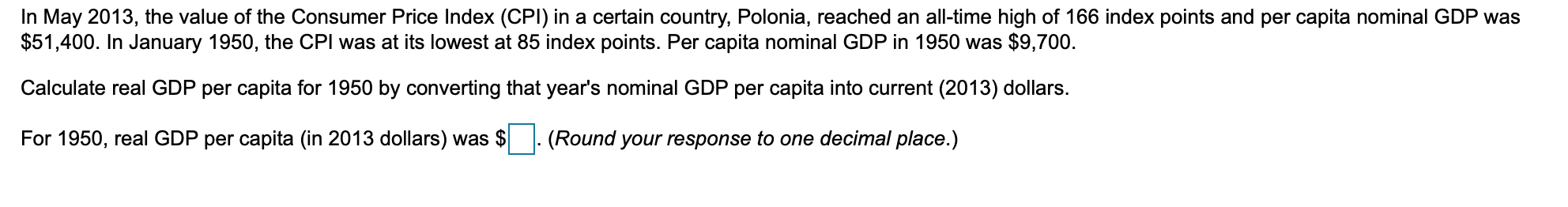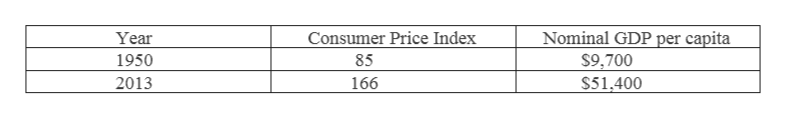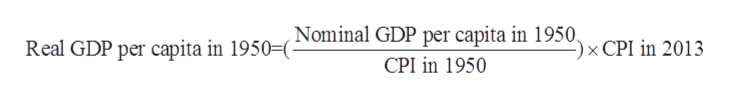In May 2013, the value of the Consumer Price Index (CPI) in a certain country, Polonia, reached an all-time high of 166 index points and per capita nominal GDP was\$51,400. In January 1950, the CPI was at its lowest at 85 index points. Per capita nominal GDP in 1950 was \$9,700.Calculate real GDP per capita for 1950 by converting that year's nominal GDP per capita into current (2013) dollars.For 1950, real GDP per capita (in 2013 dollars) was(Round your response to one decimal place.)

Question

For​ 1950, real GDP per capita​ (in 2013​ dollars) was___usd?help_outlineImage TranscriptioncloseIn May 2013, the value of the Consumer Price Index (CPI) in a certain country, Polonia, reached an all-time high of 166 index points and per capita nominal GDP was \$51,400. In January 1950, the CPI was at its lowest at 85 index points. Per capita nominal GDP in 1950 was \$9,700. Calculate real GDP per capita for 1950 by converting that year's nominal GDP per capita into current (2013) dollars. For 1950, real GDP per capita (in 2013 dollars) was (Round your response to one decimal place.) fullscreen
Step 1

The consumer price index measures the weighted average of prices of household goods and services like food, furniture etc. Nominal GDP per capita is nominal GDP divided by total population. And real GDP per capita is real GDP divided by total population.

Step 2

Given information:help_outlineImage TranscriptioncloseNominal GDP per capita Year Consumer Price Index 1950 85 \$9,700 2013 166 \$51,400 fullscreen
Step 3

Real GDP per capita for 1950 by converting the same year’s nominal GD...help_outlineImage TranscriptioncloseReal GDP per capita in 1950=(Nominal GDP per capita in 1950. -)x CPI in 2013 CPI in 1950 fullscreen

Want to see the full answer?

See Solution

Want to see this answer and more?

Our solutions are written by experts, many with advanced degrees, and available 24/7

See Solution
Tagged in